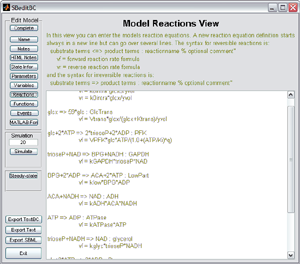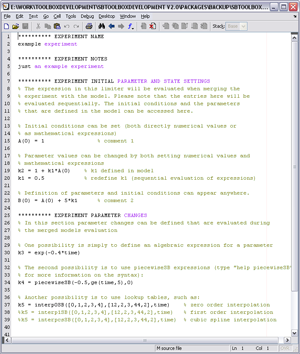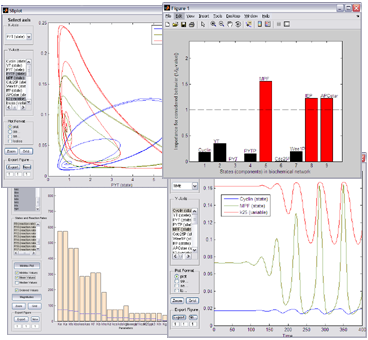# Overview and Features of SBTOOLBOX2

The Systems Biology Toolbox 2 (SBTOOLBOX2) for MATLAB offers systems biologists a powerful, open, and user extensible environment, in which to build models of biological systems. Experiments can be performed on models, just like in real lab life but in silico. The representation of models, experiments, and measurement data is intuitive and easy to use. The toolbox features a wide variety of specialized analysis tools and MATLAB adds to that by a large number of inbuilt functions and a high level scripting language, allowing the user to quickly and efficiently add new functionality.

## Main Features SBTOOLBOX2

• Representation of models, measurement data, experiment descriptions
• SBML import/export
• Deterministic and stochastic simulation
• Visualization
• Metabolic control analysis
• Stoichiometric analysis
• Parameter sensitivity analysis (Local, Global, Customizable metric, Oscillating systems)
• Determination of moiety conservations
• Localization of mechanisms leading to complex behaviors
• Statistical functions
• Signal processing functions
• Over 15 powerful global and local optimization algorithms
• Linear and quadratic programming solvers

## Model RepresentationDefine your models using a simple syntax based on differential equations or based on biochemical reaction equations. To see examples you can click on the figures to the right.

Additionally it is possible to define differential equations, initial conditions, variables, and reactions using a vectorized syntax. An example for which this syntax is useful is:

d/dt x[n] = kon*x*x[n-1] + koff*x[n+1] - (kon*x+koff)*x[n]

### SBML Enabled

The toolbox supports the import and export of SBML models. On this page you can find information about its SBML compatibility.

## Experiment RepresentationIn order to match the in silico implementation to the real workflow in a lab the toolbox allows to describe in silico experiments that are to be performed on the model. Click on the figure to the left to see an example experiment description.

Experiments and models can then be merged to obtain a simulatable model that reflects the defined experiment. Especially in the case where several different experiments are performed on the same system it is very useful to be able to only keep one copy of the model and to code the different experiments per se.

## Measurement Representation

Measurement data collected during experiments can be imported to the toolbox, visualized, manipulated. Both Excel and comma separated value (CSV) formats are accepted.

## Simulation, Analysis, etc.The toolbox features simulation (both deterministic and stochastic) and a wide range of analysis tools, such as:
• Stoichiometry
• Parameter sensitivity analysis
• Determination of moiety conservations
• Localization of mechanisms leading to complex behaviors
• Statistics (clustering, percentiles, boxplots, etc.)
• Global and local optimization algorithms
• etc.